﻿ 多目标优化的微电网运行模式

# 多目标优化的微电网运行模式Operation Mode of Multi-Energies Complementary Microgrid

Abstract: A multi-energies complementary microgrid and its mathematical model were presented, composed of gas turbine, photovoltaic, wind power generation, energy storage, and cooling/heating/electrical loads. The operation mode of microgrid was analyzed from dimensions of technology, economy, energy efficiency and environment. Combined with practical engineering cases, 5 operation modes were designed for the multi-energy-sources complementary microgrid, including low-carbon mode, economical mode, high-efficiency mode, autonomic mode, and optimized mode. The results show that these operation modes can meet the different operational requirements of micro energy network and can be extended.

1. 引言

2. 多能互补微电网模型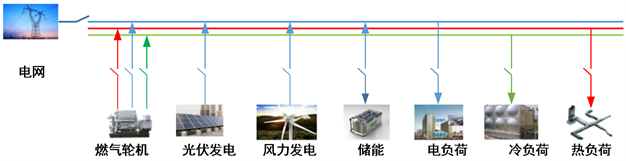Figure 1. Architecture of multi energy complementary microgrid system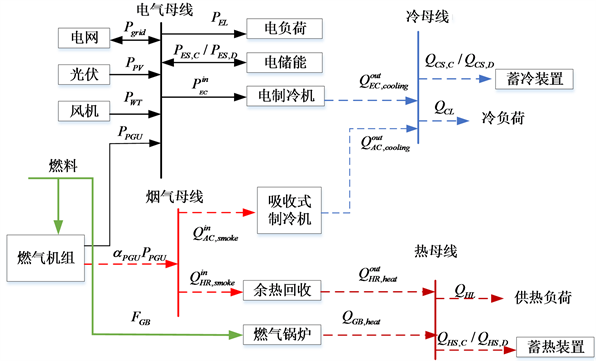Figure 2. Microgrid structure

1) 电母线平衡方程：

${P}_{grid}+{P}_{PGU}+{P}_{WT}+{P}_{PV}+{P}_{ES,D}={P}_{EL}+{P}_{EC}^{in}+{P}_{ES,C}$ (1)

2) 烟气母线平衡方程式：

${\alpha }_{PGU}{P}_{PGU}={Q}_{AC,smoke}^{in}+{Q}_{HR,smoke}^{in}$ (2)

3) 热母线平衡方程式：

${Q}_{HR,heat}^{out}+{Q}_{GB,heat}+{Q}_{HS,D}={Q}_{HL}+{Q}_{HS,C}$ (3)

4) 冷母线平衡方程：

${Q}_{EC,cooling}^{out}+{Q}_{AC,cooling}^{out}+{Q}_{CS,D}={Q}_{CS,C}+{Q}_{CL}$ (4)

3. 微电网运行策略Figure 3. Microgrid operation strategy

3.1. 技术

$\underset{P,u}{Min}\underset{i=1}{\overset{T}{\sum }}\left\{{P}_{dbuy}^{t}-{P}_{dsell}^{t}\right\}$ (5)

3.2. 经济

$\underset{P,u}{Min}\underset{t=1}{\overset{T}{\sum }}\left\{\underset{i=1}{\overset{{N}_{g}}{\sum }}\left({f}_{i,g}^{t}+{f}_{i,OM}^{t}+{f}_{i,DEP}^{t}\right)+{f}_{dbuy}^{t}-{f}_{dsell}^{t}\right\}$ (6)

3.3. 环境

$\underset{P,u}{Min}\underset{t=1}{\overset{T}{\sum }}\left\{\underset{i=1}{\overset{{N}_{g}}{\sum }}{E}_{i}^{m,t}\left({P}_{i}\right)+{E}_{buy}^{m,t}\left({P}_{i}\right)\right\}$ (7)

3.4. 能效

$\eta =\frac{3.6W+{Q}_{1}+{Q}_{2}}{B{Q}_{L}}\ast 100%$ (8)

3.5. 综合优化

$\underset{P,u}{Min}\left(\alpha {f}_{operztion}+\beta {E}_{emission}+\gamma {P}_{inter}+\delta \eta \right)$ (9)

4. 微电网运行模式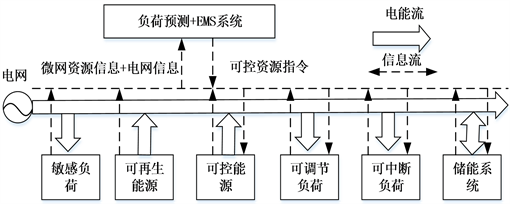Figure 4. Microgrid control diagramFigure 5. Microgrid optimal control model

4.1. 自治模式

4.2. 低碳模式

4.3. 经济模式

4.4. 能效模式

4.5. 优化模式

4.6. 模式间切换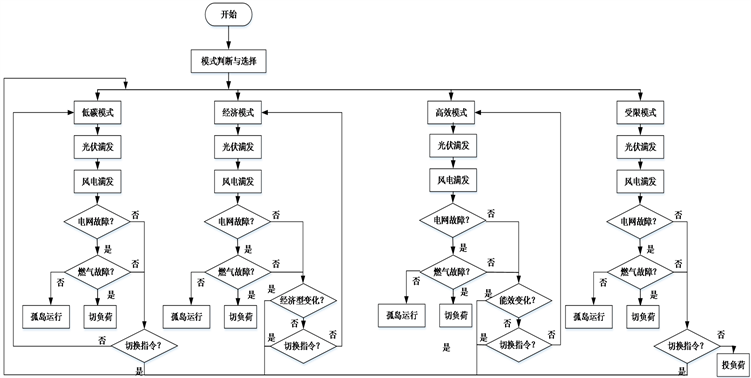Figure 6. Flow chart of operation mode conversion

5. 案例分析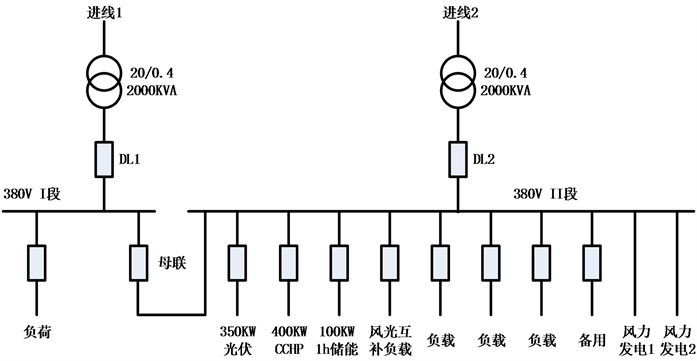Figure 7. System wiring diagram

6. 结论

 梁亮, 李建林, 惠东. 光伏-储能联合发电系统运行机理及控制策略[J]. 电力自动化设备, 2011, 31(8): 20-23.

 朱兰, 严正, 杨秀, 符杨, 陈洁. 风光储微网系统蓄电池容量优化配置方法研究[J]. 电网技术, 2012, 36(12): 26-31.

 吴杰康, 唐利涛, 黄奂, 卢永文. 基于遗传算法和数据包络分析法的水火电力系统发电多目标经济调度[J]. 电网技术, 2011, 35(5): 76-81.

 Hawkes, A.D. and Leach, M.A. (2007) Cost-Effective Operating Strategy for Residential Micro-Combined Heat and Power. Energy, 32, 711-723.
https://doi.org/10.1016/j.energy.2006.06.001

 Mago, P.J., Fumo, N. and Chamra, L.M. (2007) Methodology to Perform a Non-Conventional Evaluation of Cooling, Heating and Power Systems. Proceedings of the Institution of Mechanical Engineers, Part A: Journal of Power and Energy, 221, 1075-1087.
https://doi.org/10.1243%2F09576509JPE442

 荆有印, 白鹤, 张建良. 太阳能冷热电联供系统的多目标优化设计与运行策略分析[J]. 中国电机工程学报, 2012, 32(20): 82-87.

 Pruitt, K.A., Braun, R.J. and Newman, A.M. (2013) Establishing Conditions for the Economic Viability of Fuel Cell-Based, Combined Heat and Power Distributed Generation Systems. Applied Energy, 111, 904-920.
https://doi.org/10.1016/j.apenergy.2013.06.025

 Fang, F., Wang, Q.H. and Shi, Y. (2012) A Novel Optimal Operational Strategy for the CCHP System Based on Two Operating Modes. IEEE Transactions on Power Systems, 27, 1032-1041.
https://doi.org/10.1109/TPWRS.2011.2175490

 Flores, R.J., Shaffer, B.P. and Brouwer, J. (2014) Dynamic Distributed Generation Dispatch Strategy for Lowering the Cost of Building Energy. Applied Energy, 123, 196-208.
https://doi.org/10.1016/j.apenergy.2014.02.028

 牛铭, 黄伟, 郭佳欢, 苏玲. 微网并网时的经济运行研究[J]. 电网技术, 2010, 34(11): 38-42.

 Fumo, N., Mago, P.J. and Chamra, L.M. (2009) Emission Operational Strategy for Combined Cooling, Heating, and Power Systems. Applied Energy, 86, 2344-2350.
https://doi.org/10.1016/j.apenergy.2009.03.007

 黎灿兵, 刘屿, 曹一家, 谭益, 薛晨, 唐升卫. 低碳发电调度与节能发电调度的一致性评估[J]. 中国电机工程学报, 2011, 31(31): 94-101.

 王晓晶, 陈星莺, 陈楷, 丁孝华, 蒋宇, 余昆. 智能配电网清洁性评估指标研究[J]. 中国电机工程学报, 2013, 33(31): 43-50.

 郭力, 许东, 王成山, 王守相. 冷电联供分布式供能系统能量优化管理[J]. 电力系统自动化, 2009, 33(19): 96-100.

 吴雄, 王秀丽, 王建学, 别朝红. 微网经济调度问题的混合整数规划方法[J]. 中国电机工程学报, 2013, 33(28): 1-8.

 刘小平, 丁明, 张颖媛, 徐宁舟. 微网系统的动态经济调度[J]. 中国电机工程学报, 2011, 31(31) : 77-84.

 杨佩佩, 艾欣, 崔明勇, 雷之力. 基于粒子群优化算法的含多种供能系统的微网经济运行分析[J]. 电网技术, 2009, 33(20): 38-42.

 王成山, 洪博文, 郭力, 张德举, 刘文建. 冷热电联供微网优化调度通用建模方法[J]. 中国电机工程学报, 2013, 33(1): 26-33.

 许昌, 李旻, 袁媛, 郭苏, 刘德有, 郑源. 塔式太阳能集热-燃用天然气燃气轮机复合发电系统建模[J]. 中国电机工程学报, 2013, 33(32): 62-69.

 Ghadimi, P., Kara, S. and Kornfeld, B. (2014) The Optimal Selection of On-Site CHP Systems through Integrated Sizing and Operational Strategy. Applied Energy, 126, 38-46.
https://doi.org/10.1016/j.apenergy.2014.03.085

 王锐, 顾伟, 吴志. 含可再生能源的热电联供型微网经济运行优化[J]. 电力系统自动化, 2011, 35(8): 22-27.

 周任军, 李绍金, 陈瑞先, 李红英, 杨雨薇, 陈云. 采用模糊自修正粒子群算法的碳排放权交易冷热电多目标调度[J]. 中国电机工程学报, 2014, 34(34): 6119-6126.

Top